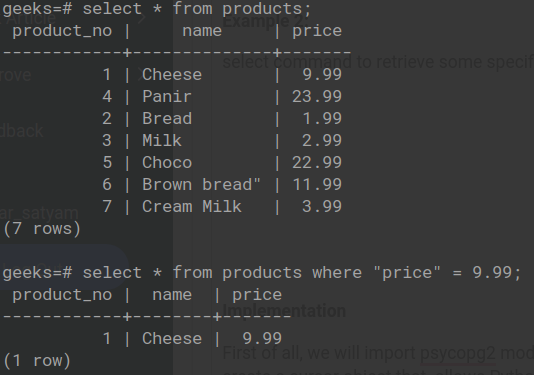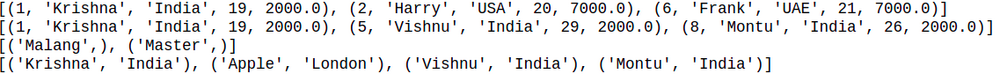GFG App
Open AppBrowser
Continue

# Python PostgreSQL – Where Clause

In this article, we are going to see how to use the Where clause in PostgreSQL using Psycopg2 in Python.

Where Clauses help us to easily deal with the databases. As we know we have a huge amount of data stored in our database, so extracting only useful and required information clauses is helpful. The WHERE clause is used to extract only those records that fulfill a required condition.

Syntax: SELECT column1, column2, ….. FROM table_name WHERE condition

#### Table demonstration with where clause:Example: At first, here we have shown how to create a table and then to insert values in it.

## Python3

 `import` `psycopg2`   `# establishing the connection` `conn ``=` `psycopg2.connect(` `    ``database``=``"test"``,` `    ``user``=``'postgres'``,` `    ``password``=``'password'``,` `    ``host``=``'localhost'``,` `    ``port``=``'5432'` `)`   `# Creating a cursor object using the cursor() method` `cursor ``=` `conn.cursor()`   `sql ``=` `'''CREATE TABLE WORKER(` `   ``ID BIGSERIAL NOT NULL PRIMARY KEY,` `   ``NAME VARCHAR(100) NOT NULL,` `   ``COUNTRY VARCHAR(50) NOT NULL,` `   ``AGE INT,` `   ``SALARY FLOAT    ` `)'''` `cursor.execute(sql)`   `# Inserting values into the table` `insert_stmt ``=` `"INSERT INTO WORKER (NAME, COUNTRY, AGE, SALARY) \` `VALUES (``%``s, ``%``s, ``%``s, ``%``s)"` `data ``=` `[(``'Krishna'``, ``'India'``, ``19``, ``2000``),` `        ``(``'Harry'``, ``'USA'``, ``20``, ``7000``),` `        ``(``'Malang'``, ``'Nepal'``, ``25``, ``5000``),` `        ``(``'Apple'``, ``'London'``, ``26``, ``2000``),` `        ``(``'Vishnu'``, ``'India'``, ``29``, ``2000``),` `        ``(``'Frank'``, ``'UAE'``, ``21``, ``7000``),` `        ``(``'Master'``, ``'USA'``, ``25``, ``5000``),` `        ``(``'Montu'``, ``'India'``, ``26``, ``2000``),` `        ``]` `cursor.executemany(insert_stmt, data)`   `# Retrieving specific records using the where clause` `cursor.execute(``"SELECT * from WORKER WHERE AGE <23"``)` `print``(cursor.fetchall())`   `# Retrieving specific records using the where clause` `cursor.execute(``"SELECT * from WORKER WHERE COUNTRY='India' "``)` `print``(cursor.fetchall())`     `# Retrieving name of employees whose salary is 5000` `cursor.execute(``"SELECT name from WORKER WHERE salary=5000 "``)` `print``(cursor.fetchall())`   `# Retrieving name and country of employees whose salary is 2000` `cursor.execute(``"SELECT name, country from WORKER WHERE salary=2000 "``)` `print``(cursor.fetchall())`   `# Commit your changes in the database` `conn.commit()`   `# Closing the connection` `conn.close()`

Output:where clause using pyscopg2 in Python

My Personal Notes arrow_drop_up